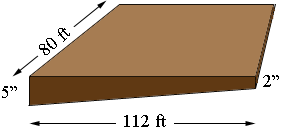SEARCH HOMEMath Central Quandaries & QueriesQuestion from Carlos: I have a lot with a slope. On the right I need to bring it up 2', From that point to the left is 112' which at this point I need to bring it up 5' for it to be level . The distance front to back is 80'. What is the cubic yards of this area that I need to fill in.Hi Carlos,

I drew a rough sketch, not to scaleThe volume of fill is the area of the cross section times the length of 80 fet. The cross section is a trapezoid and the area of a trapezoid is the average of the lengths of the parallel sides times the distance between the parallel sides. I want all the dimensions in feet so the parallel sides are of length $\large \frac{5}{12}$ feet and $\large \frac{2}{12}.$ Hence the volume of fill required is

$\frac12 \left( \frac{5}{12} + \frac{2}{12} \right) \times 112 \times 80 \mbox{ cubic feet.}$

There are 27 cubic feet in a cubic yard so divide the volume in cubic feet by 27 to obtain the volume in cubic yards.

HarleyMath Central is supported by the University of Regina and The Pacific Institute for the Mathematical Sciences.